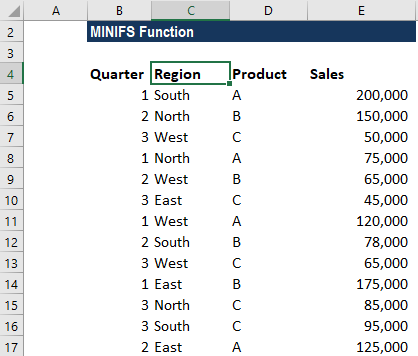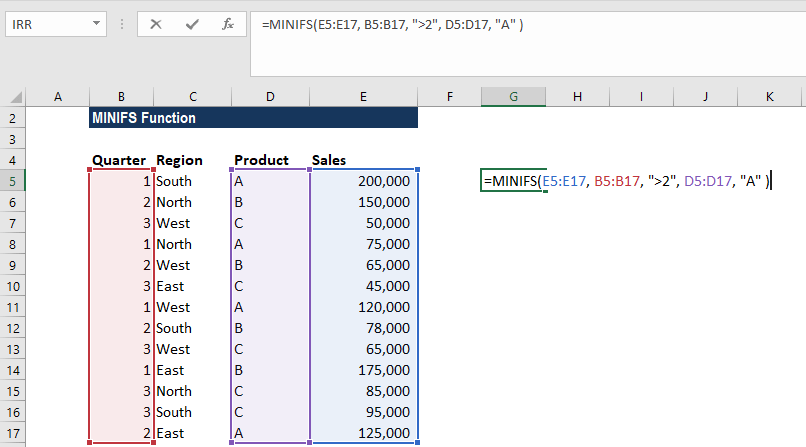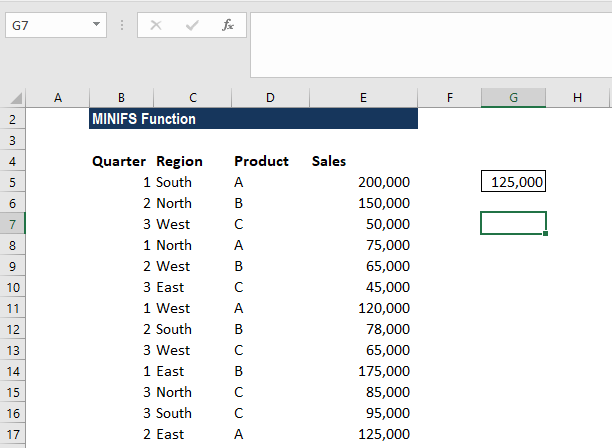# MINIFS Function

Returns the smallest numeric value that meets one or more criteria in a given range of values

## What is the MINIFS Function?

The MINIFS function is categorized under Excel Statistical functions. It will return the smallest numeric value that meets one or more criteria in a given range of values.

In financial analysis, the MINIFS function can be used to extract a minimum numeric value. For example, we can get the minimum value of test scores by a male and a female.

### Formula

=MINIFS(min_range, criteria_range1, criteria1, [criteria_range2, criteria2], …)

The MINIFS function uses the following arguments:

1. Min_range (required argument) – This is the actual range of cells in which the minimum value will be determined.
2. Criteria_range1 (required argument) – The set of cells to evaluate with the criteria.
3. Criteria1 (required argument) – This is the criteria in the form of a number, expression, or text that defines which cells will be evaluated as the minimum.
4. Criteria_range2, criteria2, … (optional arguments) – This is the additional array(s) of values to be tested and the respective conditions to test.

MINIFS supports logical operators (>, <, <>, =) and wildcards (*, ?) for partial matching. It can be used with criteria based on dates, numbers, text, and other conditions.

### How to use the MINIFS Function in Excel?

To understand the uses of the MINIFS function, let’s look at an example:

#### Example 1

Suppose we are given the sales figure for 3 products from 3 regions, as shown below:The formula to be used to find the sales figures for product A in Q2 would be:We get the result below:### A few notes about the MINIFS Function:

1. #VALUE! error – Occurs if the min_range and criteria_range arrays are not of equal lengths.
2. #NAME? error – Occurs when we are using a pre-2016 Excel version that doesn’t support the function.
3. The MINIFS function is not case-sensitive. For example, when comparing the values in the criteria_range against the criteria, the text strings “TEXT” and “text” will be considered to be a match.
4. The function can handle up to 126 pairs of criteria_range and criteria arguments.

Thanks for reading CFI’s guide to the Excel MINIFS function. By taking the time to learn and master these functions, you’ll significantly speed up your financial analysis. To learn more, check out these additional CFI resources: